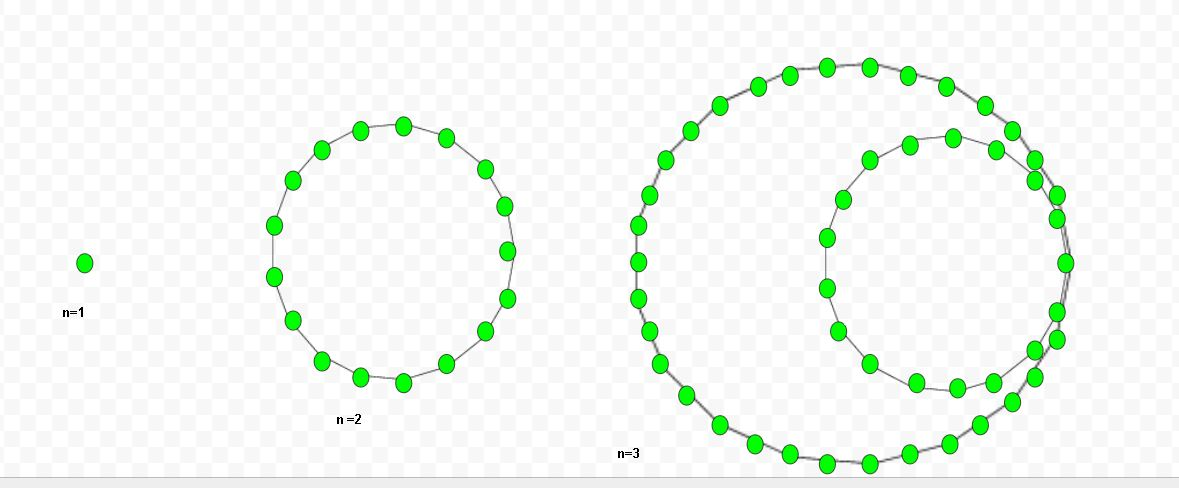Given a number n, the task is to find the nth heptadecagonal number .
A heptadecagonal number is class of figurate number. It has seventeen sided polygon called heptadecagon. The n-th heptadecagonal number count’s the seventeen number of dots and all others dots are surrounding with a common sharing corner and make a pattern.

Examples:

Input : 5
Output :155

Input :9
Output :549Formula to calculate nth heptadecagonal number:## C++

 // C++ program to find Nth  // heptadecagonal number  #include  using namespace std;     // Function to calculate heptadecagonal  // number  int heptadecagonalNum(long int n)  {      return ((15 * n * n) - 13 * n) / 2;  }     // Driver Code  int main()  {      long int n = 3;      cout << n << "th Heptadecagonal number : ";      cout << heptadecagonalNum(n);      cout << endl;      n = 8;      cout << n << "th Heptadecagonal number : ";      cout << heptadecagonalNum(n);         return 0;  }

## Java

 // Java program to find Nth heptadecagonal number  import java.io.*;     class GFG {             // Function to calculate heptadecagonal      // number      static long heptadecagonalNum(long n)      {          return ((15 * n * n) - 13 * n) / 2;      }             // Driver Code      public static void main (String[] args)      {          long n = 3;          System.out.print( n + "th Heptadecagonal"                                   + " number : ");          System.out.println( heptadecagonalNum(n));                     n = 8;          System.out.print( n + "th Heptadecagonal"                                  + " number : ");          System.out.print( heptadecagonalNum(n));      }  }     // This code is contributed by anuj_67.

## Python3

 # Python program to find Nth  # heptadecagonal number     # Function to calculate  # heptadecagonal number  def heptadecagonalNum(n):         # Formula to calculate nth      # heptadecagonal number      return ((15 * n * n) - 13 * n) // 2       # Driver Code  n = 3 print("%sth Heptadecagonal number : " %n,                      heptadecagonalNum(n))  n = 8 print("%sth Heptadecagonal number: " %n,                      heptadecagonalNum(n))                                                # This code is contributed by ajit

## C#

 // C# program to find Nth   // heptadecagonal number  using System;  class GFG {             // Function to calculate       // heptadecagonal number      static long heptadecagonalNum(long n)      {          return ((15 * n * n) -                     13 * n) / 2;      }             // Driver Code      public static void Main ()      {          long n = 3;          Console.Write( n + "th Heptadecagonal"                                 + " number : ");          Console.WriteLine( heptadecagonalNum(n));                     n = 8;          Console.Write( n + "th Heptadecagonal"                                 + " number : ");          Console.WriteLine( heptadecagonalNum(n));      }  }     // This code is contributed by anuj_67.

## PHP

 

Output

3th Heptadecagonal number : 48


Attention reader! Don’t stop learning now. Get hold of all the important DSA concepts with the DSA Self Paced Course at a student-friendly price and become industry ready.

My Personal Notes arrow_drop_upCheck out this Author's contributed articles.

If you like GeeksforGeeks and would like to contribute, you can also write an article using contribute.geeksforgeeks.org or mail your article to contribute@geeksforgeeks.org. See your article appearing on the GeeksforGeeks main page and help other Geeks.

Please Improve this article if you find anything incorrect by clicking on the "Improve Article" button below.

Improved By : vt_m, jit_t